Mathematical and Physical Journal
for High Schools
Issued by the MATFUND Foundation
 Already signed up? New to KöMaL?

# KöMaL Problems in Physics, May 2012

Show/hide problems of signs:## Problems with sign 'M'

Deadline expired on June 11, 2012.

M. 324. Measure the coefficient of kinetic friction between the tyre of a bicycle wheel and the dry asphalt.

(6 pont)

statistics## Problems with sign 'P'

Deadline expired on June 11, 2012.

P. 4444. The distance between two town is exactly 60 km along the highway. Some part of the highway goes through inhabitated area, where the speed limit is 50 km/h. In the uninhabitated area the speed limit is 90 km/h. If the speed limits are kept the shortest time to get from one of the town to the other is 46.6 minutes. What is the ratio of the lengths of the highway which is in the uninhabitated and in the inhabitated regions?

(3 pont)

solution (in Hungarian), statistics

P. 4445. The figure shows a right circular cone of uniform density and of height 56 cm. Where is the centre of mass of that truncated cone which is gained if the original cone is cut into two at the midpoint of its height, and the top part of the cone is removed?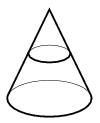(3 pont)

solution (in Hungarian), statistics

P. 4446. A slow neutron collides with a stationary one, such that the angle between the direction of the original motion and the direction of the neutron's motion is. In which direction does the stationary neutron begin to move after the collision?

(4 pont)

solution (in Hungarian), statistics

P. 4447. What are the resistances of the resistors R1 and R2, shown in the figure, if the voltage supply is 40 V, and the power dissipated on resistor R is 80 W, the readings on the ammeter and on the voltmeter are 3 A and 30 V, respectively.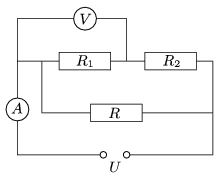(4 pont)

solution (in Hungarian), statistics

P. 4448. The speed of sound in carbon dioxide is 1.3 times as much as that of in air. Two children, sitting 2 and 2 metres from the opposite sides of a balloon which is filled with carbon dioxide, and hanged in the height of the heads of the children, found that the balloon reinforce'' their whisper the most, exactly at this position.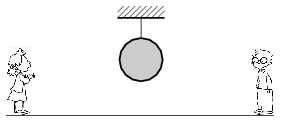Estimate the diameter of the balloon.

(5 pont)

solution (in Hungarian), statistics

P. 4449. A space probe is to be shot from a point of the interplanetarial space at some speed with respect to the Sun, which is exactly half the speed at which the object would have circular path through the same point.

a) With respect to the Sun into which direction must the space probe be launched if it is to move away from the starting point the least?

b) With respect to the Sun into which direction must the space probe be launched if it is to move away from the starting point the most?

c) By what factor will the greatest distance between the probe and the starting point be greater in case of b) than in case of a)?

(Any effects except for the gravitation of the Sun are negligible.)

(5 pont)

solution (in Hungarian), statistics

P. 4450. The amount of water vapour in the air of the wind reaching a mountain is 12 g/m3, the temperature of air is 20 oC. Along the slope of the hill the ascending air cools down, and half of its water vapour content precipitates as rain. Estimate the temperature of the air descending on the other side of the mountain.

(5 pont)

solution (in Hungarian), statistics

P. 4451. A sample of diatomic, ideal gas is taken through a cyclic process. The cyclic process can be composed of such open processes that the specific heat capacity of the gas during the certain processes does not change. The cyclic process is shown in the graph of the figure. The algebraic sum of the absorbed and delivered heat by the gas up to an instantaneous state, (Q), is shown on the horizontal axis, and the total amount of work done by the gas on its environment up to reaching the certain instantaneous state, (W*), is shown on the y axis.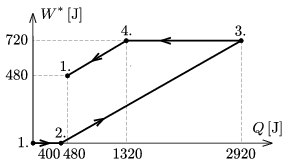a) Using the graph, determine the efficiency of the cyclic process.

b) What types of processes build up the cyclic process? Reason your statements.

c) Let the pressure of the gas at state 1 be p0, and its volume be V0. Using these parameters plot the cyclic process on the p-V diagram.

(5 pont)

solution (in Hungarian), statistics

P. 4452. In a long thin iron rod uniformly changing magnetic induction can be created. The induced voltage in a wire around the rod is U=10 mV. A wire is put around the rod such that into one half of it a resistor of resistance R1=10 k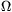, and into the other half a resistor of resistance R2=20 kare inserted.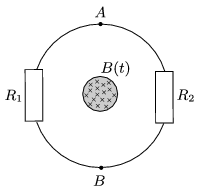a) What is the reading on the ideal voltmeter connected to the A and B terminals of the resistors?

b) What would be the reading on a voltmeter of resistance Rm=100 k?

Does the measured voltage depend on to which side of the rod the meter is placed?

(5 pont)

solution (in Hungarian), statistics

P. 4453. The density of the energy of the electric field inside a straight cylinder shaped metal rod is constant, its magnitude isJ/m3; the density of the energy of the magnetic field inside the rod at a distance of r=2 cm from the symmetry axis of the rod isJ/m3.

a) What is the resistivity of the material of the rod?

b) What kind of metal was the rod made of?

(5 pont)

solution (in Hungarian), statistics

P. 4454. The volume of a cubic aquarium, which is filled with water halfway, is V=8 litres. Salt is carefully dissolved in the water such that the refractive index of the water, with respect to light which illuminates the water from below, changes with the height h measured from the bottom of the aquarium as a function of n(h)=n0-kh2, where n0=1.35 is the refractive index at the bottom and k=2 m-2 is a constant.

The aquarium is illuminated by a light-beam perpendicularly to one of its side. On the other side a screen is moved. The screen always remains parallel to that side of the aquarium which is illuminated by the light. How far is the screen from the aquarium, when only a thin sharp, horizontal line of light can be observed on it?

(6 pont)

solution (in Hungarian), statistics